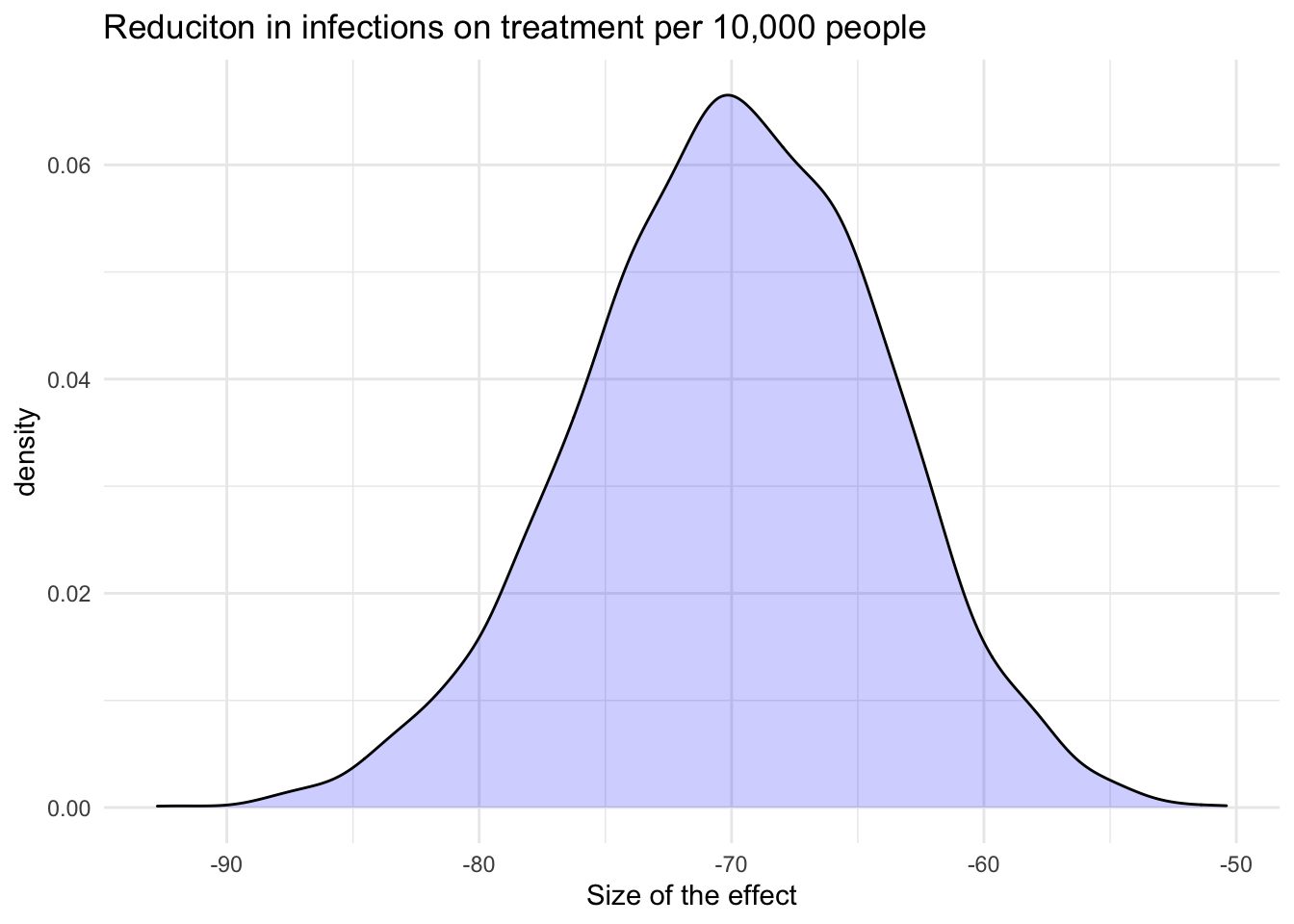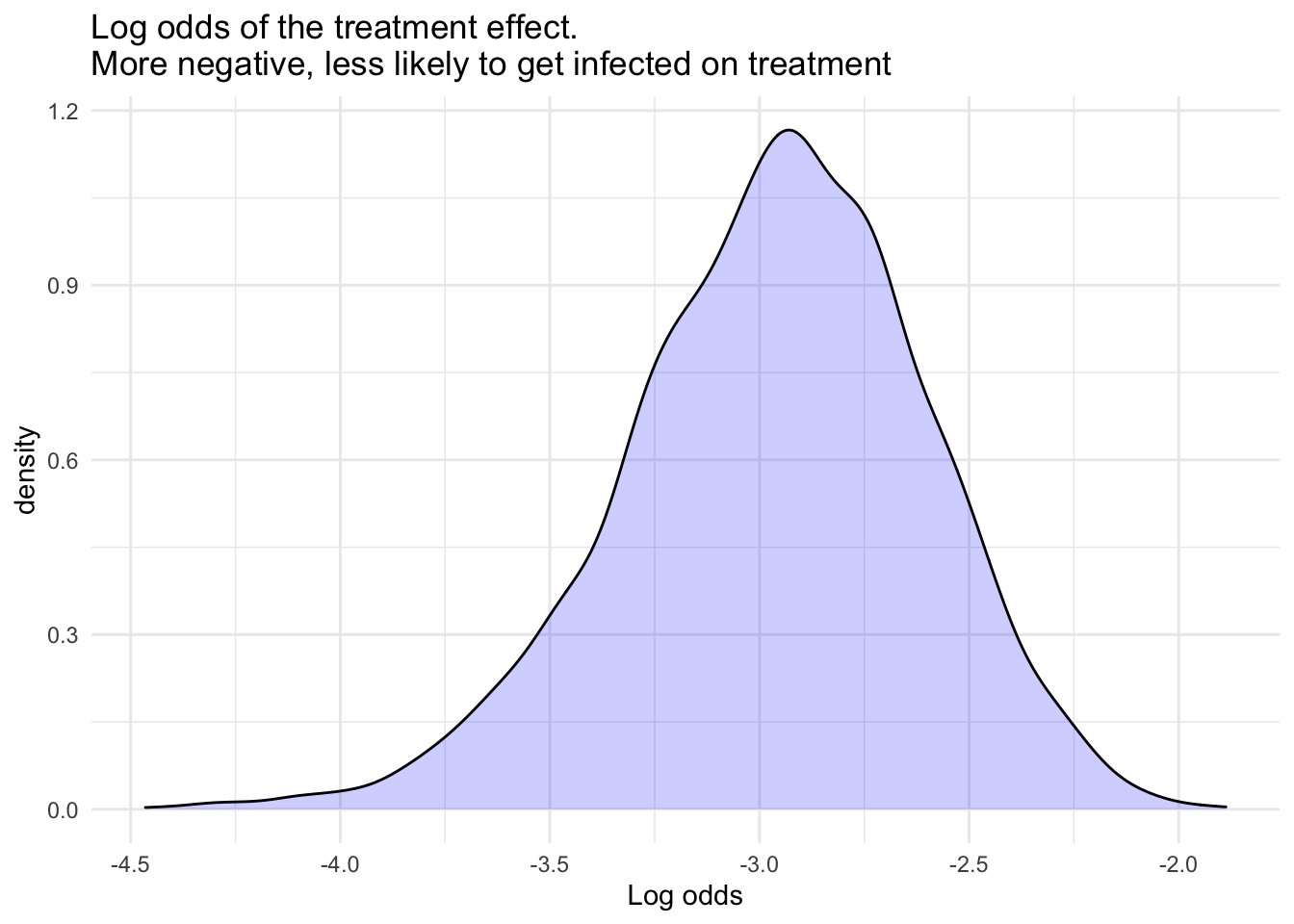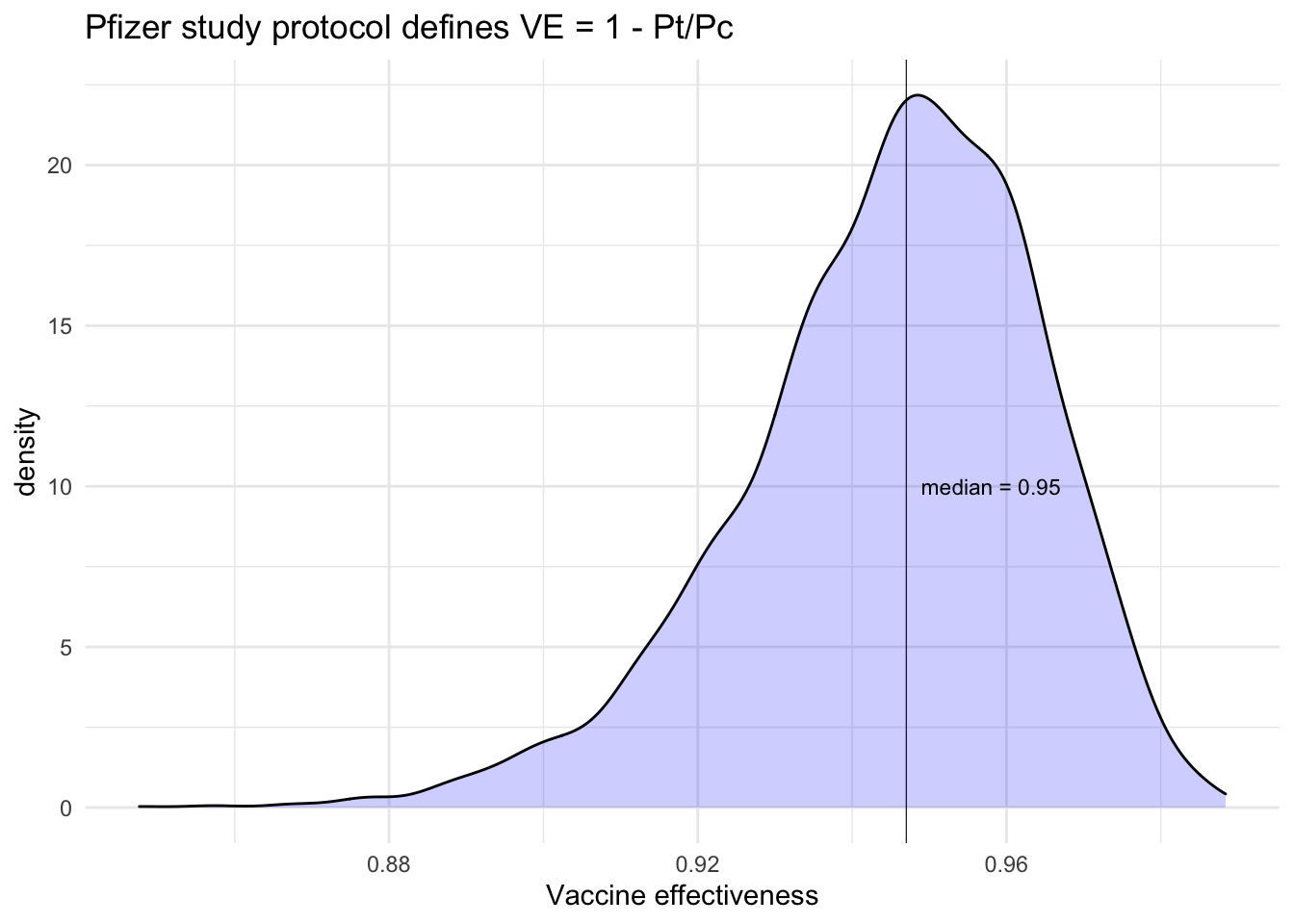## Assumptions

From the New York Times: New Pfizer Results: Coronavirus Vaccine Is Safe and 95% Effective.

n <- 4.4e4  # number of volunteers
r_c <- 162  # number of events in control
r_t <- 8    # number of events in vaccine group

NYT reports a 44 thousand person trial with half of the people going to treatment and half to control. They further report that 162 people developed COVID in the control group and 8 were in the vaccine group.

What is the probability that the vaccine is effective and what is the uncertainty in that probability? The Pfizer protocol defines vaccine effectiveness as follows:

$\text{VE} = 1 - \frac{p_{t}}{p_{c}}$ Here $$p_{t}$$ is infection rate in vaccinated group and $$p_{c}$$ is the rate in the control group.

## Model

Also, let’s assume that we have no prior beliefs about the effectiveness rate and so our model is as follows: \begin{align} p_{c} \sim \textsf{beta}(1, 1) \\ p_{t} \sim \textsf{beta}(1, 1) \\ y_{c} \sim \textsf{binomial}(n_{c},p_{c}) \\ y_{t} \sim \textsf{binomial}(n_{t},p_{t}) \\ \end{align} The treatment effect and $$VE$$ can be computed directly from this model.

\begin{align} \text{effect} = p_{t} - p_{c} \\ \text{VE} = 1 - \frac{p_{t}}{p_{c}} \end{align}

The effect will have a distribution and to get the probability of the effect (this is different from VE), we sum up the negative area. For this problem we do not need Stan, but I am including it here to show how easy it is to specify this model, once we write down the math above.

data {
int<lower=1> r_c; // num events, control
int<lower=1> r_t; // num events, treatment
int<lower=1> n_c; // num cases, control
int<lower=1> n_t; // num cases, treatment
int<lower=1> a;   // prior a for beta(a, b)
int<lower=1> b;   // prior b for beta(a, b)
}
parameters {
real<lower=0, upper=1> p_c; // binomial p for control
real<lower=0, upper=1> p_t; // binomial p for treatment
}
model {
p_c ~ beta(a, b); // prior for control
p_t ~ beta(a, b); // prior for treatment
r_c ~ binomial(n_c, p_c); // likelihood for control
r_t ~ binomial(n_t, p_t); // likelihood for treatment
}
generated quantities {
real effect   = p_t - p_c;      // treatment effect
real VE       = 1 - p_t / p_c;  // vaccine effectiveness
real log_odds = log(p_t / (1 - p_t)) -
log(p_c / (1 - p_c));
}

## Running the model

Let’s run this model from R and make a few plots.

library(cmdstanr)
library(posterior)
library(ggplot2)

# first we get the data ready for Stan
d <- list(r_c = r_c, r_t = r_t, n_c = n/2,
n_t = n/2, a = 1, b = 1) # beta(1,1) -> uniform prior

# compile the model
mod <- cmdstan_model("vaccine.stan")
## Model executable is up to date!
# fit the model with MCMC
fit <- mod$sample( data = d, seed = 123, chains = 4, parallel_chains = 4, refresh = 0 ) ## Running MCMC with 4 parallel chains... ## ## Chain 1 finished in 0.2 seconds. ## Chain 2 finished in 0.2 seconds. ## Chain 3 finished in 0.2 seconds. ## Chain 4 finished in 0.2 seconds. ## ## All 4 chains finished successfully. ## Mean chain execution time: 0.2 seconds. ## Total execution time: 0.3 seconds. # parameter summary fit$summary()
## # A tibble: 6 x 10
##   variable     mean   median      sd     mad       q5      q95  rhat ess_bulk
##   <chr>       <dbl>    <dbl>   <dbl>   <dbl>    <dbl>    <dbl> <dbl>    <dbl>
## 1 lp__     -1.04e+3 -1.04e+3 1.03e+0 7.04e-1 -1.04e+3 -1.04e+3  1.00    1645.
## 2 p_c       7.40e-3  7.40e-3 5.78e-4 5.86e-4  6.48e-3  8.40e-3  1.00    3209.
## 3 p_t       4.06e-4  3.91e-4 1.37e-4 1.32e-4  2.08e-4  6.54e-4  1.00    3137.
## 4 effect   -7.00e-3 -6.99e-3 5.95e-4 6.03e-4 -8.01e-3 -6.05e-3  1.00    3217.
## 5 VE        9.45e-1  9.47e-1 1.92e-2 1.84e-2  9.10e-1  9.72e-1  1.00    3124.
## 6 log_odds -2.97e+0 -2.95e+0 3.59e-1 3.51e-1 -3.59e+0 -2.42e+0  1.00    3123.
## # … with 1 more variable: ess_tail <dbl>
# extract the draws
draws <- fit$draws() # Convert to data frame draws <- posterior::as_draws_df(draws) head(draws) ## # A draws_df: 6 iterations, 1 chains, and 6 variables ## lp__ p_c p_t effect VE log_odds ## 1 -1041 0.0072 0.00045 -0.0068 0.94 -2.8 ## 2 -1041 0.0075 0.00034 -0.0071 0.95 -3.1 ## 3 -1044 0.0084 0.00072 -0.0077 0.91 -2.5 ## 4 -1043 0.0065 0.00027 -0.0063 0.96 -3.2 ## 5 -1043 0.0068 0.00026 -0.0065 0.96 -3.2 ## 6 -1042 0.0078 0.00029 -0.0075 0.96 -3.3 ## # ... hidden meta-columns {'.chain', '.iteration', '.draw'} # look at the distribution of the effect ggplot(draws, aes(x = effect*1e4)) + geom_density(fill = "blue", alpha = .2) + expand_limits(y = 0) + theme_minimal() + xlab("Size of the effect") + ggtitle("Reduciton in infections on treatment per 10,000 people")# Probability that there is an effect is the negative mass of the effect # distribution; more negative favors treatment -- there is no question # that there is an effect round(mean(draws$effect < 0), 2)
##  1
ggplot(draws, aes(x = log_odds)) +
geom_density(fill = "blue", alpha = .2) +
expand_limits(y = 0) + theme_minimal() +
xlab("Log odds") +
ggtitle("Log odds of the treatment effect.
More negative, less likely to get infected on treatment")label_txt <- paste("median =", round(median(draws$VE), 2)) ggplot(draws, aes(x = VE)) + geom_density(fill = "blue", alpha = .2) + expand_limits(y = 0) + theme_minimal() + geom_vline(xintercept = median(draws$VE), size = 0.2) +
annotate("text", x = 0.958, y = 10, label = label_txt, size = 3) +
xlab("Vaccine effectiveness") +
ggtitle("Pfizer study protocol defines VE = 1 - Pt/Pc")quant <- round(quantile(draws\$VE, probs = c(0.05, 0.5, 0.95)), 2)

So if we observe 162 infections in placebo group and 8 in the vaccinated group, the vaccine could be considered to be about 0.95 effective with the likely effectiveness anywhere between 0.91 and 0.97 which represents a median and 90% quantile interval. We should insist that reporters produce uncertainty estimates and the reporters in turn should insist that companies provide them.